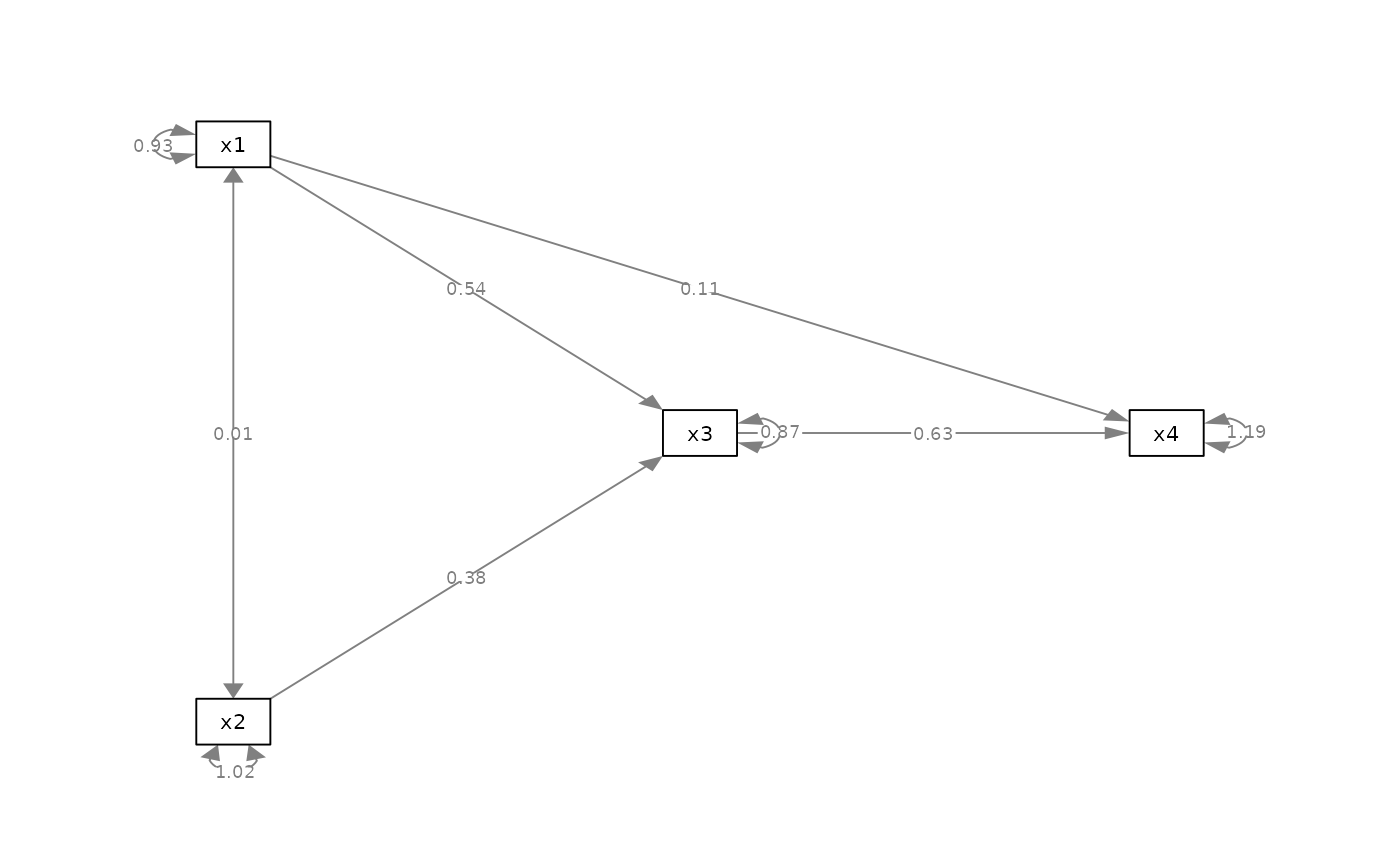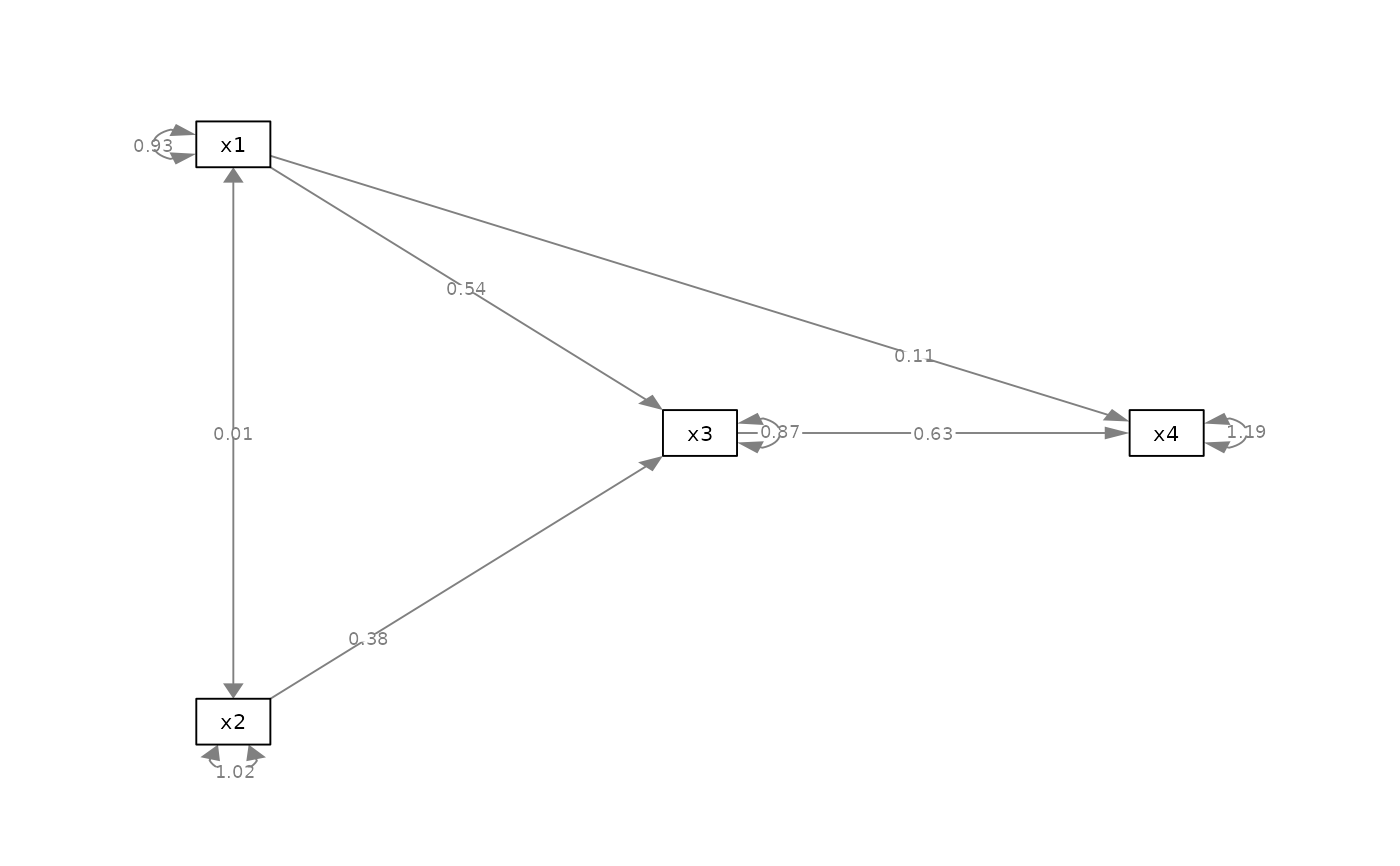Set the positions of edge labels of selected edges.

## Usage

set_edge_label_position(semPaths_plot, position_list = NULL)

## Arguments

semPaths_plot

A qgraph::qgraph object generated by semPlot::semPaths, or a similar qgraph object modified by other semptools functions.

position_list

A named vector or a list of named lists. For a named vector, the name of an element should be the path as specified by lavaan::model.syntax or as appeared in lavaan::parameterEstimates(). For example, to change position of the edge label of the path regressing y on x, the name should be "y ~ x". The value is the position. The mid-point of the edge is 0.5. The closer the value to 1, the closer the label to the left-hand-side node (y in this example). The closer the value to 0, the close the label to the right-hand-side node (x in this example). For example, c("y ~ x1" = .2, "y ~ x2" = .7) moves the path coefficient from x1 to y closer to x, and the path coefficient from x2 to y closer to y. For a list of named lists, each named list should have three named values: from, to, and new_position. The edge label position of the edge from from to to will be set to new_position. For example, list(list(from = "x1", to = "y", new_position = .2), list(from = "x2", to = "y", new_position = .7)) is equivalent to the named vector above.

## Value

A qgraph::qgraph based on the original one, with edge label positions for selected edges changed.

## Details

Modify a qgraph::qgraph object generated by semPlot::semPaths and change the edge label positions of selected edges.

## Examples

mod_pa <-
'x1 ~~ x2
x3 ~  x1 + x2
x4 ~  x1 + x3
'
fit_pa <- lavaan::sem(mod_pa, pa_example)
lavaan::parameterEstimates(fit_pa)[, c("lhs", "op", "rhs", "est", "pvalue")]
#>   lhs op rhs   est pvalue
#> 1  x1 ~~  x2 0.005  0.957
#> 2  x3  ~  x1 0.537  0.000
#> 3  x3  ~  x2 0.376  0.000
#> 4  x4  ~  x1 0.111  0.382
#> 5  x4  ~  x3 0.629  0.000
#> 6  x3 ~~  x3 0.874  0.000
#> 7  x4 ~~  x4 1.194  0.000
#> 8  x1 ~~  x1 0.933  0.000
#> 9  x2 ~~  x2 1.017  0.000
m <- matrix(c("x1",   NA,   NA,
NA, "x3", "x4",
"x2",   NA,   NA), byrow = TRUE, 3, 3)
p_pa <- semPlot::semPaths(fit_pa, whatLabels="est",
style = "ram",
nCharNodes = 0, nCharEdges = 0,
layout = m)my_position_vector <- c("x3 ~ x2" = .25,
"x4 ~ x1" = .75)
p_pa2v <- set_edge_label_position(p_pa, my_position_vector)
plot(p_pa2v)my_position_list <- list(list(from = "x2", to = "x3", new_position =  .25),
list(from = "x1", to = "x4", new_position =  .75))
p_pa2l <- set_edge_label_position(p_pa, my_position_list)
plot(p_pa2l)